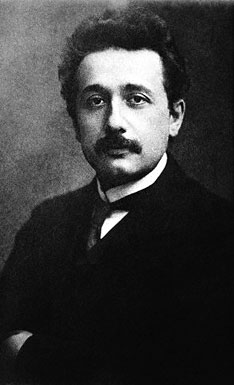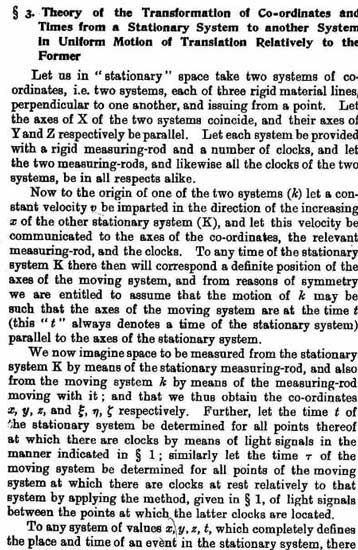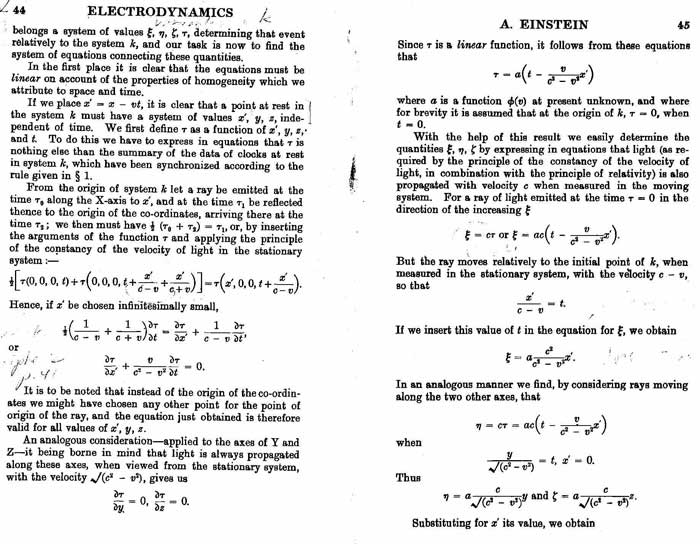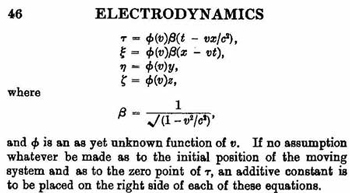go to homepage A Mathematical Critiqueof Einstein's 1905 paperby Miles Mathis First written January 2002 In this chapter I will critique Einstein's original paper of 1905—probably the most famous physics paper of all time (in 1944 a manuscript was purchased for \$6 million—who knows what it would fetch now). I have copied the 2 and 1/2 pages of equations that lead up to gamma here, so that you can follow along, line for line.The first part of the article is just an introduction to the problem, which I will not repeat here. It is perhaps better to approach the problem fresh, anyway. It is much simpler than history has made it. In short, Einstein has one important postulate to add to Newton and Galileo. It is this:"Any ray of light moves in the stationary system of co-ordinates with the determined velocity c, whether the ray be admitted by a stationary or moving body." This is just a restatement of the constancy of c, but it is a precise wording of it, and it will come up very soon in his math. That is why I quote it in full. It is the second of two "basic principles" of relativity. The first principle is that systems or bodies in "uniform translatory motion" relative to eachother have the same laws. That is, neither one is primary: either may be taken as "at rest." This is not so much a postulate as it is an hypothesis (as Einstein admits in this paper) but since I agree with the intent of both principles, it does not matter here.I will touch on one point before moving on. Principle 2, quoted above, already contains a confusion of terms. A moving body requires a second co-ordinate system—that is why Einstein invents multiple systems to begin with: to explain the motion. But the second part of principle 2 ignores this fact. He says, "whether the ray be admitted by a stationary or moving body." But, obviously, if the ray is admitted by a moving body, it is not in "the stationary system of co-ordinates." It is in the moving system of co-ordinates. A better statement of principle 2 would have been that light is measured to travel c, from any and all co-ordinate systems.Very soon after this Einstein introduces his two co-ordinate systems, which in this paper he calls K and k, where K is the stationary system. Then he gives us the variables, x, y, z, and t in K; and ξ, η, ζ and τ in k. In short, given x and t, he wants to find a transformation term that will express ξ and τ [ ξ and τ were replaced by x' and t' in later algebraic derivations, such as the one in his book Relativity]. The last thing we are given is a velocity v. This, Einstein tells us, is the velocity of the entire system k in "the direction of the increasing x of K" But, I ask, is this the velocity of k as measured from k, or as measured from K? Einstein does not tell us, though it is absolutely crucial that we know. K and k each have their own clocks and measuring rods, which are not equivalent (by the rules of Relativity). They will therefore each measure velocity differently. In fact, they will measure the velocity of k differently. But Einstein does not assign v to either system. This is Einstein's greatest error in the whole derivation of gamma. Since v is unprimed and un-Greek, you may assume it is k as measured from K. But if this is true, then the velocity of k as measured by k must have a value too. Notice that Einstein never once, in this or any other derivation, creates a variable for that v, nor does he discover an equation which yields it. [The velocity we get in later equations—the velocity of the current v tranformation—is the velocity of an object moving within k. It is not the velocity of k itself.]Then we get the equation x' = x - vt. Einstein says,"If we place x' = x - vt, it is clear that a point at rest in the system k must have a system of values x', y, z independent of time. We first define τ as a function of x', y, z and t. To do this we have to express in equations that τ is nothing else than the summary of the data of clocks at rest in system k, which have been synchronized. . ."So, he states outright that x', y, and z are now the set of variables "at rest in the system k." But he has already assigned y and z to K. A point at rest relative to k is moving relative to K, since k is moving relative to K. So "at rest in the system k" is not equivalent to "at rest in K". This being true, it also means that ξ, η, and ζ have just been bumped up into a third system—the system that is moving in k—which he has no label for. But x' is a variable with two allegiances: he says it is now in k, but by the equation x' = x - vt it is still connected to K. Unless he means for x and v to also be "at rest in k", in which case we might as well do away with K altogether—it has become superfluous. Likewise, the variable t is also a dual citizen. Einstein has it variously in K and k. When it is with x', it is in k. When it is with x, it is in K. Tau (τ) is in the undefined third co-ordinate system, with ξ, η, and ζ. Notice especially that Einstein has created an x' but no t'. He has three x variables but not three t variables.He then says, "From the origin of system k let a ray be emitted at the time τ0 along the x-axis to x', and at the time τ1 be reflected thence to the origin of the co-ordinates, arriving there at the time τ2." So the ray takes the place of the man in the train. Einstein has a movement within a movement here: k is moving in K, and the lightray is moving in k. This provides two degrees of relativity, which Einstein is trying to calculate at once. But he does not seem to realize that he is doing this. He doesn't have enough variables to do it successfully, so his variables keep sliding from K to k. He needs three sets of variables and three co-ordinate systems.The other mistake is to use a light ray for his object moving in k. Principle 2 states that light is measured c, whether from a moving or stationary system. This makes light a special case. It is not like a man on a train. c, unlike v or v', does not change with position of measurement. This is Einstein's second greatest error in this paper.Next he prepares for a bit of calculus—that is why he is talking of functions. He doesn't need calculus here, but it is the only way he can get the reader more confused. He gives us this strange equation: 1/2[τ(0,0,0,t) + τ(0,0,0, t + x'/(c - v) + x'/(c + v))] = τ(x', 0,0, t + x'/(c - v)) This is a preparatory step to differentiation, but we don't need to go there since this equation is faulty. It is supposed to be an expansion of1/2(τ0 + τ2) = τ1 But the corresponding time at τ1 in either k or K is not t + x'/(c - v) . First of all, this violates Einstein's own Principle 2, which I quoted in full above. The speed of light must be measured the same from anywhere. But Einstein himself is subtracting v from it! Before giving us a partial equation for τ, supposedly from all his differentiation, he tells us that "light is always propagated along these axes [Y and Z], when viewed from the stationary system, with the velocity √(c2 - v2)." All that, as an aside, almost as a footnote. He gives us no equations to show why light should move like that along the Y and Z axes. Where did that square root come from? One can only suppose he borrowed it from somewhere, most likely Lorentz. But it just stands there, naked, with nothing leading into it and nothing leading out. Obviously it is supposed to be the old Pythagorean way of comparing the two lightrays in the interferometer, that I have already critiqued. But we don't have two light rays here. He has given us one lightray going up and back, in the x-direction of both K and k. So his equation for Y and Z is false—he has given us v as the velocity in the x-direction. We have no velocity in the Y or Z direction, not in K or k. What is more astonishing is the fact that he again violates Principle 2. He says, "when viewed from the stationary system." But Principle 2 reads, "Any ray of light moves in the stationary system of co-ordinates with the determined velocity c, whether the ray be admitted by a stationary or moving body." k is that moving body. Finally, Einstein gives us this equation: τ = a[t - vx'/(c2 - v2)] where "a is a function of v at present unknown."Then he gives us thisξ = cτ This is the equation that becomes x' = ct' in the book Relativity. I have already shown that it is false [in several other papers], since time dilating implies a larger τ, not a smaller. But by substituting this false equation into the previous one we get,ξ = ac[t - vx'/(c2 - v2)]Then Einstein says that t = x'/(c - v), which I have just shown is wrong. But he substitutes it into the above equation.After simplifying he gets,ξ = ac2x'/(c2 - v2)then he substitutes x - vt for x', which yields, ξ = ac2(x - vt)/(c2 - v2)Then, as you can see, Einstein gives us some more nonsensical equations for η and ζ. Being in the y and z directions, they can have nothing to do with v, which Einstein told us at the top of p. 43 was "in the direction of the increasing x of K."But we are basically finished. c2/(c2 - v2) is our transformation term. Einstein calls it β here, but it has come to be known as γ. He never had a β variable in any equation, but it suddenly appears at the end as the thing we were looking for all along. The variable "a" has been lost to the dustbin in all the rewritings of these equations, with no explanation that I have ever seen. Einstein's final error is a simple mathematical one. He assumes that c2/(c2 - v2) = 1/√(1 - v 2 /c2) When in fact it is simply1/(1 – v2/c2) There is no square root!Either he reduced the equation wrong, or he has some hidden math steps here he is not sharing with us. It may be that he thinks that those last y and z equations will affect his transformation term β somehow. But in his rewriting of the math in later books, he does not assume that y and z affect calculations in the x-direction. Nor does anyone who parrots this derivation of gamma. But if you think about it, the only way to get a Pythagorean square root into a linear problem in one direction, is to mess it up in just this way—to assume, for some reason, that an x-velocity is affected by y and z. One final point. Einstein later changes the math of Special Relativity. It has been said that he did this to simplfy it for general audiences, specifically for the book Relativity. Those who say this also point to the fact that he changed the math to make it even more difficult after he completed General Relativity. For, at that time, he imported the tensor calculus used in General Relativity to express Special Relativity. In his lectures at Princeton he used this very difficult math. He had two derivations of Special Relativity working after 1916: one a revamped algebraic derivation (in the appendix of Relativity) that is very similar to the one I showed in this paper, the other a tensor calculus derivation.But both changes mask the basic errors of the original paper—they do not fix them. In the algebraic solution, he gets rid of the Greek letters. Unfortunately he replaces ξ with x'. But they are far from equivalent. x' is the x-variable in a non-moving k, as he states above. ξ is the x-variable moving within k. It is the x-variable that belongs to the lightray, which is moving. Nor does he fix the equation x' = ct'. It is false whether the x-variable is ξ or x'.The tensor calculus derivation also takes all the givens of the paper of 1905 as still given. It fixes none of them. All it does is make the contradictions harder to see. There is absolutely no reason to use tensor calculus to solve the problem of Special Relativity, as Einstein presents it and as it stands to this day. It does not even call for regular calculus, as Einstein proved in the appendix of his book. A problem should be solved with the simplest math that will solve it—especially a problem of applied math. This keeps the concepts and assumptions as near to the surface as possible, where they may ride out the light of day.Now let us look at Einstein's derivation of the equation for the addition of velocities. In his 1905 paper he did not differentiate his x equation in order to find his relative velocity equation, like they do now in textbooks. He simply combined his equations algebraicly, like this:From earlier in the paper Einstein found:γ = gamma = 1 /√(1 - v2/c2) τ = γ(t - vx/c2)ξ = γ(x - vt)Now he says, if a point is moving in k, let ξ = wτ where w "is a constant".Notice two things. One, Einstein now has a point moving in k instead of a light ray. He seems to have recognized his earlier mistake, and he changes his moving object from a light ray to a point. This is really odd. If I am right and he noticed the theoretical difference between the light ray and the point, then why didn't he go back and correct the first part of the derivation. On the other hand, if I am wrong and he didn't see his mistake, why did he make the basic change? You don't normally change your objects in the middle of a derivation. Two, he does not define this new velocity variable at all, beyond saying it is a constant. This is really extraordinary. One must look at the sentences two or three times, to be sure that he intends w to be the velocity variable. He does not fully define w, because he can't. If he states outright that it is a velocity variable, then he must assign it to one of his co-ordinate systems. Very soon you will see why that would be fatal to him.By substitution, he gets,wτ = γ(x - vt)wγ(t - vx/c2) = γ(x - vt)wt - wvx/c2 = x - vtx + wvx/c2 = wt + vtx(1 + wv/c2) = (w + v)tx =     ( w + v )t         1 + wv/c2Now, watch this last step very closely. He reduces the above equation to:V =       w + v           1 + wv/c2This is the current value for V. This equation stands to this day.But to reduce like he did he must assume that V = x/tWe have been given that w = ξ/τSo what does v equal? v is what x over what t? V = x/tw = ξ/τv = ?/?You may say, well maybe the x is x'. Maybe, but what is the t? He has no third t-variable anywhere, in this paper. And in later derivations, when he does have a t', he has no τ. He never has three t variables. What we need to solve for an addition of velocities, amazingly enough, is four t-variables and five x variables.t0 = the time of K from Kt = the time of the point as seen from Kt' = the time of k as seen from Kt'' = the time of k as seen from k τ = the time of the point as seen from k.τ' = the time of the point as seen from the pointbut t" = τ' = t0 We need five x-variablesx = displacement of the point, as measured by Kx' = displacement of k, as measured by Kx" = displacement of k as measured by k.ξ = displacement of the point, as measured by kξ' = displacement of the point as measured by the pointEinstein says that v is the velocity of k relative to K. w is the velocity of a point relative to k. V is the velocity of that point relative to K.but to solve we also need,w' = velocity of the point measured by the pointv' = velocity of k as measured by kw' = ξ'/t 0 v' = x"/t0τ = t0 + (ξ'/c)w = ξ'/[t0 + (ξ'/c)] = w'/(1 + w'/c)w' = w/(1 - w/c) v = x"/t't' = t0 + (x"/c)v = x"/[t0 + (x"/c)] = v'/(1 + v'/c)v' = v/[1 - (v/c)]t = t0 + (ξ' + x")/cV = (ξ' + x")/t = (ξ' + x")/[t0 + (ξ' + x")/c]Multiply the last equation by 1/t0//1/t0{Eq. 5}  V    =       v' + w'                          1 + [(v' + w')/c] V =                     v            +             w                          1 - (v/c)                    1 - (w/c)                             v             +             w                 1 +      1 - (v/c)                    1 - (w/c)                                        c     =              v + w - (2vw/c)           1 - v/c - w/c + vw/c2 + [v + w - (2vw/c)]/c      =      v + w - (2vw/c)                    1 - vw/c2 Just to be sure that gamma does not apply to the transformation of two degrees for t, let us find τ' in terms of v and t, like Einstein did.t = t0 + (ξ' + x")/cw' = ξ'/t0v' = x"/t0t = t0 + (w't0+ v't0)/cw' = w/[1 – (w/c)] and v' = v/[1 – (v/c)]t0 = τ't = τ' + wτ'/[c(1 - w/c)] + vτ'/[c(1 - v/c])t = τ'{1 + [w/c(1 - w/c)] + v/c(1 - v/c)}t/τ' = (c - v)(c + v)     +      w/(c - v)      +      v/(c - w)           (c - v)(c - w)           (c - v)(c - w)           (c - v)(c - w)     = c2 - wv           =          1 - wv/c2         (c - w)(c - v)                (1 - w/c)(1 - v/c)Similar, but not gamma. Not surprising, since gamma only has one velocity variable. Notice that in Einstein's derivation of gamma, regarding x and t, he already had two velocities. His set-up for the addition of velocity section is exactly the same as his set-up for x and t, in the first section. The only difference is he had a light ray moving—as his second velocity—in the first part, and a point in the second part. But in both sections he is seeking equations for two degrees of relativity. Now, you may say, why not use "equation 5", in the section on V above? It looks very much like Einstein's equation, except that we are adding the velocities in the denominator rather than multiplying them. At most speeds this would only be a small correction to Einstein and would seem to imply that his math was not that far off. We can't use that equation for one very important reason. The velocity variables don't match Einstein's. Mine are prime, his were not. Mine are the local velocities of k and the point. His are actually undefined, so it is impossible to say what he meant for them to stand for. But I assume he intended them to be the measured velocities of the point and k, from k and K, respectively. I assume this because he never provides us with an equation to discover the velocity of k relative to K, or the point relative to k. He doesn't derive these equations because he thought those were his givens. But now that it turns out that his final equation suggests that his givens were local velocities, measured by the moving systems themselves. And this means that the velocity of k relative to K and the velocity of the point relative to k are still unknowns. Einstein has derived no equations for first-degree relativity!The other reason not to use equation 5 is that in most real situations we will not be given the local velocities. In using the relativity equations on quanta, for instance, the givens are not local velocities. We would be given relative, or measured-from-a-distance, numbers to begin with, and would need an equation to determine the addition of these numbers. The famous experiment of Fizeau (explained by Einstein) is another example. We are given the speed of the liquid. But this is our determination of the speed of the liquid, not the liquid's. The given is not a local measurement of the system. Please notice that my new equation for the addition of velocities gives us numbers that are very close to Einstein's in most situations. It differs from his in having another easily comprehensible term in the numerator and a minus sign instead of a plus sign in the denominator. But it may be used with confidence, since it has been derived from a thoroughly analyzed situation, as above, from five different co-ordinate systems.The true transformation equation for velocity of one degree of relativity is the one I used above, in my derivation of V. v =          v'                 1 + v'/c go to homepagego to chapter 7 If this paper was useful to you in any way, please consider donating a dollar (or more) to the SAVE THE ARTISTS FOUNDATION. This will allow me to continue writing these "unpublishable" things. Don't be confused by paying Melisa Smith--that is just one of my many noms de plume. If you are a Paypal user, there is no fee; so it might be worth your while to become one. Otherwise they will rob us 33 cents for each transaction.